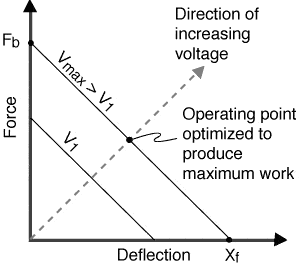# Piezoelectric transformer efficiency

Dear all,

How is the efficiency of piezoelectric transformer higher than electromechanical coupling factor?

Thank you,
Husain

berkeman
Mentor
Dear all,

How is the efficiency of piezoelectric transformer higher than electromechanical coupling factor?

Thank you,
Husain

Here is some detailed info on the piezo transformer http://www.ti.com/lit/an/slyt107/slyt107.pdf

In short, a piezoelectric transformer is a piezoelectric material that has a two sets of electrodes, a output and input set. Voltage at the mechanical resonance is introduced at the input. The entire device vibrates. The piezoelectric material between the output electrodes also vibrates. A impedance load is placed across the output to draw power out. In effect, the whole system behaves electrically like a transformer.

The electromechanical coupling factor is the ratio between electrical energy and the mechanical energy which can be harvested from the material, and visa versa. The coupling factor is typically 0.7 or less, but they transformation of power has an efficiency of 90% plus.

Please note that the coupling factor is not a loss factor. For more details on the coupling factor see:
https://www.americanpiezo.com/knowledge-center/piezo-theory/piezoelectric-constants.html

dlgoff
Gold Member
The electromechanical coupling factor, k, is for free deflection. Here, the piezoelectric primary is constrained vertically to maximize the force on the secondary.

From http://www.piezo.com/tech2intropiezotrans.html

Free deflection (Xf) refers to displacement attained at the maximum recommended voltage level when the actuator is completely free to move and is not asked to exert any force. Blocked force (Fb) refers to the force exerted at the maximum recommended voltage level when the actuator is totally blocked and not allowed to move.My inclination for the answer is that k depends on the open circuit elastic compliance, while the short circuit compliance is used when electrically driving the ceramic and when extracting the output power.

I think I maybe asking a too specialized question.

I think I understand it. Only considering the PZT transformer, losses account for the efficiency, and k accounts for the other performance characteristics such as step up/step down voltages.

k is an energy conversion factor, not a loss conversion factor. The electrical energy not converted to mechanical energy is stored as electrical energy. In the case of the oscillator/piezo transformer, power in=power out is conserved except for the losses. If k was the efficiency, then the electrical oscillation power would need to continuously increase, which is wrong because of power conservation.